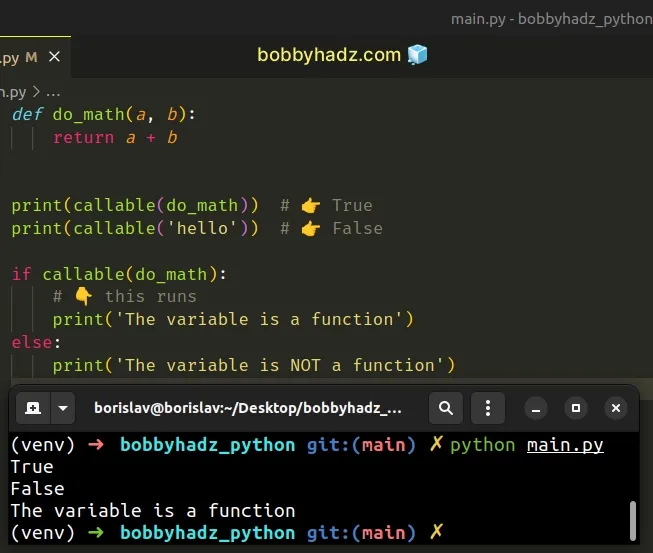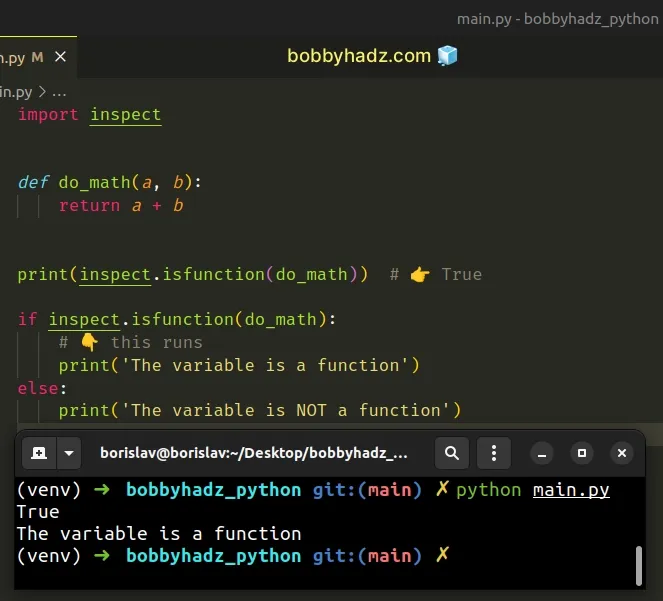# How to check if a variable is a Function in PythonLast updated: Feb 22, 2023
3 min## #Check if a variable is a Function in Python

Use the `callable()` function to check if a variable is a function, e.g. `if callable(function):`.

The `callable()` function takes an object and returns `True` if the object is callable, otherwise, `False` is returned.

main.py
```Copied!```def do_math(a, b):
return a + b

print(callable(do_math))  # 👉️ True
print(callable('hello'))  # 👉️ False

if callable(do_math):
# 👇️ this runs
print('The variable is a function')
else:
print('The variable is NOT a function')
``````We used the `callable()` built-in function to check if a variable is a function.

The callable function takes an object as an argument and returns `True` if the object appears callable, otherwise `False` is returned.

main.py
```Copied!```print(callable(lambda x: x * 2))  # 👉️ True
print(callable(3.14))  # 👉️ False
``````

If the `callable()` function returns `True`, it is still possible that calling the object fails, however, if it returns `False`, calling the object will never succeed.

Note that the `callable()` function doesn't check if the passed-in value is specifically a function, it checks if the value is callable.

It also returns `True` for classes.

main.py
```Copied!```class Employee:
pass

print(callable(Employee))  # 👉️ True
print(callable(int))  # 👉️ True
``````

## #Check if a variable is a Function using inspect.isfunction()

An alternative approach is to use the `inspect.isfunction()` method.

The `inspect.isfunction()` method returns `True` if the supplied object is a Python function, otherwise `False` is returned.

main.py
```Copied!```import inspect

def do_math(a, b):
return a + b

print(inspect.isfunction(do_math))  # 👉️ True

if inspect.isfunction(do_math):
# 👇️ this runs
print('The variable is a function')
else:
print('The variable is NOT a function')
``````The inspect.isfunction method takes an object and returns `True` if the object is a Python function.

The method would return `False` if passed another callable, e.g. a class.

main.py
```Copied!```import inspect

class Employee:
pass

print(inspect.isfunction(Employee))  # 👉️ False
print(inspect.isfunction(int))  # 👉️ False
``````
However, an important thing to note is that the method only returns `True` if provided a Python function.

The method returns `False` for most built-in functions because they are implemented in C, not in Python.

main.py
```Copied!```import inspect

print(inspect.isfunction(zip))  # 👉️ False
print(inspect.isfunction(map))  # 👉️ False

print(callable(zip))  # 👉️ True
print(callable(map))  # 👉️ True
``````

The `zip` and `map` functions are implemented in C, so the `inspect.isfunction()` method returned `False`.

The `callable` function works as expected because it simply checks if the provided object is callable.

## #Check if a variable is a Function using `types.FunctionType`

You might also use the `types.FunctionType` class to check if a variable is a function.

main.py
```Copied!```import types

def do_math(a, b):
return a + b

print(isinstance(do_math, types.FunctionType))  # 👉️ True

print(isinstance(zip, types.FunctionType))  # 👉️ False
print(isinstance(map, types.FunctionType))  # 👉️ False
``````

However, the class also returns `True` only for Python functions.

The isinstance function returns `True` if the passed-in object is an instance or a subclass of the passed-in class.

The `types.FunctionType` class works in the same way the `inspect.isfunction()` method does.

However, the class is a bit more indirect, so there's no good reason to use it.

## #Check if a variable is a Function using `hasattr()`

You can also use the `hasattr()` function to check if a variable is a function.

main.py
```Copied!```def do_math(a, b):
return a + b

if hasattr(do_math, '__call__'):
# 👇️ this runs
print('The variable is a function')
else:
print('The variable is NOT a function')

print(hasattr(do_math, '__call__'))  # 👉️ True
``````

The hasattr function takes the following 2 parameters:

NameDescription
`object`The object we want to test for the existence of the attribute
`name`The name of the attribute to check for in the object

The `hasattr()` function returns `True` if the string is the name of one of the object's attributes, otherwise `False` is returned.

If the object has a `__call__` attribute then it is callable.